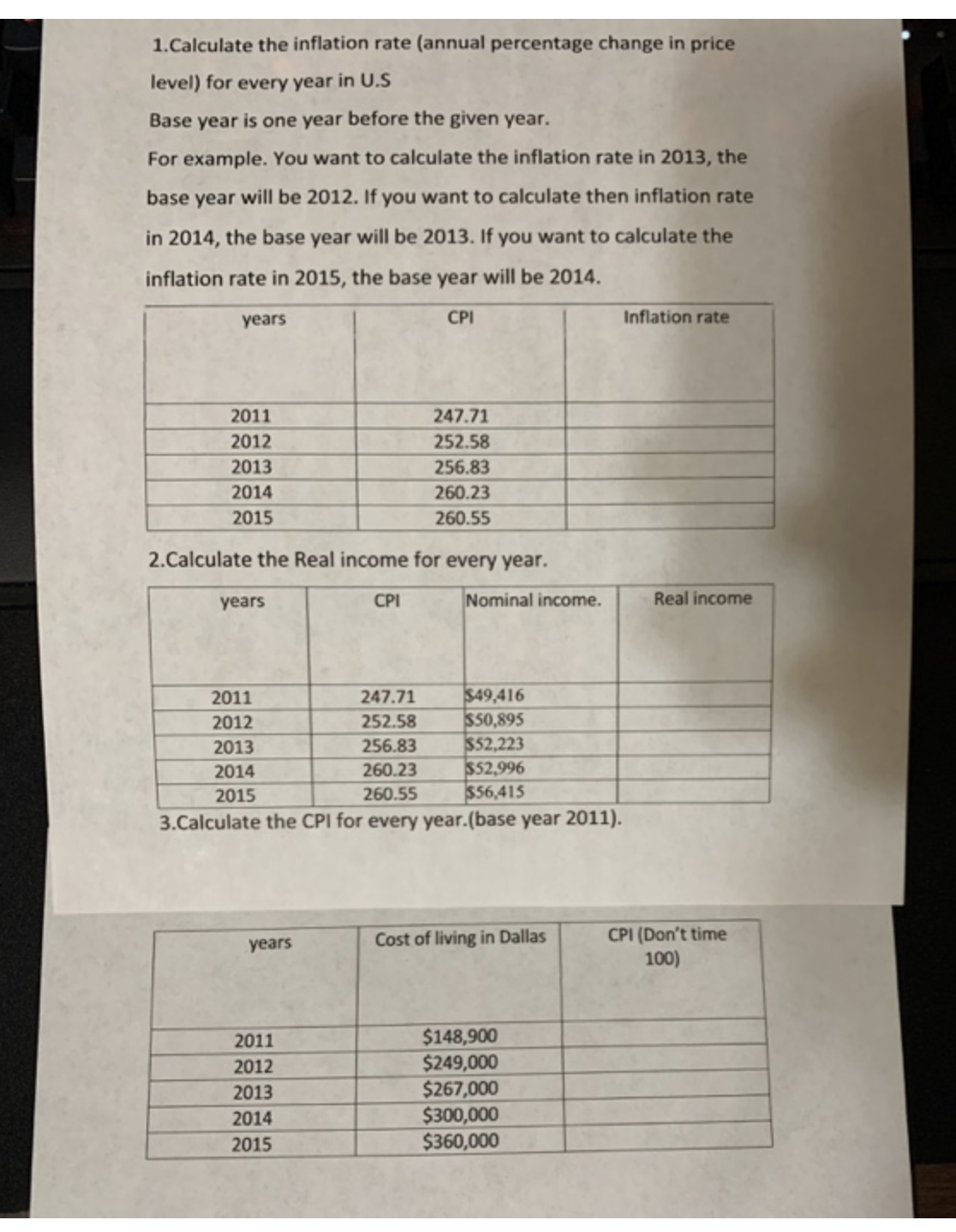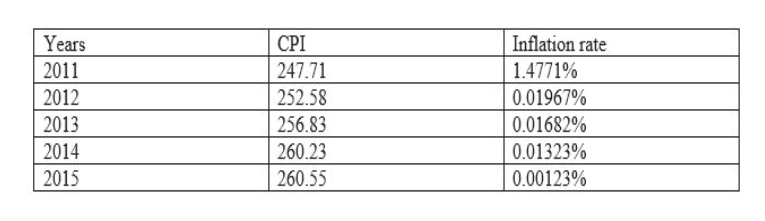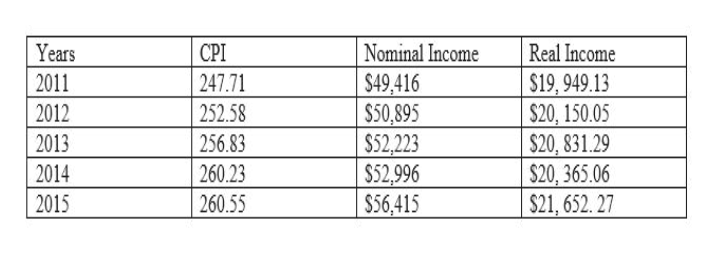# 1.Calculate the inflation rate (annual percentage change in pricelevel) for every year in U.SBase year is one year before the given year.For example. You want to calculate the inflation rate in 2013, thebase year will be 2012. If you want to calculate then inflation ratein 2014, the base year will be 2013. If you want to calculate theinflation rate in 2015, the base year will be 2014Inflation rateCPIyears2011247.71252.5820122013256.832014260.232015260.552.Calculate the Real income for every year.Nominal income.Real incomeCPIyears\$49,416\$50,895\$52,223\$52,996\$56,4152011247.71252.582012256.832013260.232014260.5520153.Calculate the CPI for every year. (base year 2011).CPI (Don't time100Cost of living in Dallasyears\$148,900\$249,000\$267,000\$300,000\$360,00020112012201320142015

Question
25 viewshelp_outlineImage Transcriptionclose1.Calculate the inflation rate (annual percentage change in price level) for every year in U.S Base year is one year before the given year. For example. You want to calculate the inflation rate in 2013, the base year will be 2012. If you want to calculate then inflation rate in 2014, the base year will be 2013. If you want to calculate the inflation rate in 2015, the base year will be 2014 Inflation rate CPI years 2011 247.71 252.58 2012 2013 256.83 2014 260.23 2015 260.55 2.Calculate the Real income for every year. Nominal income. Real income CPI years \$49,416 \$50,895 \$52,223 \$52,996 \$56,415 2011 247.71 252.58 2012 256.83 2013 260.23 2014 260.55 2015 3.Calculate the CPI for every year. (base year 2011). CPI (Don't time 100 Cost of living in Dallas years \$148,900 \$249,000 \$267,000 \$300,000 \$360,000 2011 2012 2013 2014 2015 fullscreen
check_circle

Step 1

(1)

The inflation rate can be estimated by using the following formula:

Inflation rate = (CPIn – CPIn-1) / CPIn

Where n = No. of years.

Assume year 2010 as the base year for 2011 and the value of year 2010 as 100.

Hence, the above table can be written as follows:help_outlineImage TranscriptioncloseInflation rate Years CPI 247.71 2011 14771% 2012 252.58 0.01967% 256.83 2013 0.01682% 2014 260.23 0.01323% 2015 260.55 0.00123% fullscreen
Step 2

Working notes:

Inflation rate (2011) = (247.71 -100) / 100 = 1.4771%

Inflation rate (2012) = (252.58 – 247.71) / 247.71 = 0.01967%

Inflation rate (2013) = (256.83 – 252.58) / 252.58 = 0.01682%

Inflation rate (2014) = (260.23 – 256.83) / 256.83 = 0.01323%

Inflation rate (2015) = (260.55 – 260.23) / 260.23 = 0.00123%

Step 3

(2)

We know that,

Real Income = (Nominal Income / CPI) * 100

Therefore,

Real Income (2011) = (\$49,416 / 247.71) *100 = \$19, 949.13

Real Income (2012) = (\$50,895 / 252.58) *100 = \$20, 150.05

Real Income (2013) = (\$52,223 / 256.23) *100 = \$20,...help_outlineImage TranscriptioncloseYears CPI 247.71 Nominal Income Real Income \$19,949.13 \$20, 150.05 \$20, 831.29 \$20, 365.06 \$21,652. 27 2011 \$49,416 \$50,895 \$52,223 \$52,996 \$56,415 2012 252.58 2013 256.83 260.23 2014 2015 260.55 fullscreen

### Want to see the full answer?

See Solution

#### Want to see this answer and more?

Solutions are written by subject experts who are available 24/7. Questions are typically answered within 1 hour.*

See Solution
*Response times may vary by subject and question.
Tagged in